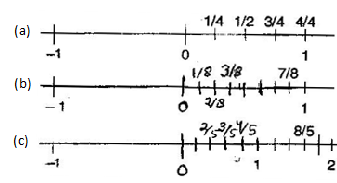1. /
2. CBSE
3. /
4. Class 06
5. /
6. Mathematics
7. /
8. NCERT Solutions for Class...

# NCERT Solutions for Class 6 Maths Exercise 7.2

NCERT solutions for Maths Fractions## NCERT Solutions for Class 6 Maths Fractions

###### Question 1.Draw number lines and locate the points on them:

(a){tex}\frac{1}{2},\frac{1}{4},\frac{3}{4},\frac{4}{4}{/tex}

(b){tex}\frac{1}{8},\frac{2}{8},\frac{3}{8},\frac{7}{8}{/tex}

(c){tex}\frac{2}{5},\frac{3}{5},\frac{8}{5},\frac{4}{5}{/tex}NCERT Solutions for Class 6 Maths Exercise 7.2

###### Question 2.Express the following fractions as mixed fractions:

(a){tex}\frac{{20}}{3}{/tex}

(b){tex}\frac{{11}}{5}{/tex}

(c){tex}\frac{{17}}{7}{/tex}

(d){tex}\frac{{28}}{5}{/tex}

(e){tex}\frac{{19}}{6}{/tex}

(f){tex}\frac{{35}}{9}{/tex}

(a)

3{tex}\mathop{\left){\vphantom{1\begin{gathered}

{\text{ }}20 \\\\\\

\underline { – 18} \\\\\\

\underline {{\text{ 2}}} \\\\\\

\end{gathered} }}\right.

\!\!\!\!\overline{\,\,\,\vphantom 1{\begin{gathered}

{\text{ }}20 \\\\\\

\underline { – 18} \\\\\\

\underline {{\text{ 2}}} \\\\\\

\end{gathered} }}}

\limits^{\displaystyle\,\,\, 6}{/tex}

(b)

5{tex}\mathop{\left){\vphantom{1\begin{gathered}

{\text{ 11}} \\\\\\

\underline { – 10} \\\\\\

\underline {{\text{ 1}}} \\\\\\

\end{gathered} }}\right.

\!\!\!\!\overline{\,\,\,\vphantom 1{\begin{gathered}

{\text{ 11}} \\\\\\

\underline { – 10} \\\\\\

\underline {{\text{ 1}}} \\\\\\

\end{gathered} }}}

\limits^{\displaystyle\,\,\, 2}{/tex}

{tex}\therefore {/tex}{tex}\frac{{20}}{3} = 6\frac{2}{3}{/tex}

{tex}\therefore {/tex}{tex}\frac{{11}}{5} = 2\frac{1}{5}{/tex}

(c)

7{tex}\mathop{\left){\vphantom{1\begin{gathered}

{\text{ 17}} \\\\\\

\underline { – 14} \\\\\\

\underline {{\text{ 3}}} \\\\\\

\end{gathered} }}\right.

\!\!\!\!\overline{\,\,\,\vphantom 1{\begin{gathered}

{\text{ 17}} \\\\\\

\underline { – 14} \\\\\\

\underline {{\text{ 3}}} \\\\\\

\end{gathered} }}}

\limits^{\displaystyle\,\,\, 2}{/tex}

(d)

5{tex}\mathop{\left){\vphantom{1\begin{gathered}

{\text{ 28}} \\\\\\

\underline { – 25} \\\\\\

\underline {{\text{ 3}}} \\\\\\

\end{gathered} }}\right.

\!\!\!\!\overline{\,\,\,\vphantom 1{\begin{gathered}

{\text{ 28}} \\\\\\

\underline { – 25} \\\\\\

\underline {{\text{ 3}}} \\\\\\

\end{gathered} }}}

\limits^{\displaystyle\,\,\, 5}{/tex}

{tex}\therefore {/tex}{tex}\frac{{17}}{7} = 2\frac{3}{7}{/tex}

{tex}\therefore {/tex}{tex}\frac{{28}}{5} = 5\frac{3}{5}{/tex}

(e)

6{tex}\mathop{\left){\vphantom{1\begin{gathered}

{\text{ 19}} \\\\\\

\underline { – 18} \\\\\\

\underline {{\text{ 1}}} \\\\\\

\end{gathered} }}\right.

\!\!\!\!\overline{\,\,\,\vphantom 1{\begin{gathered}

{\text{ 19}} \\\\\\

\underline { – 18} \\\\\\

\underline {{\text{ 1}}} \\\\\\

\end{gathered} }}}

\limits^{\displaystyle\,\,\, 3}{/tex}

(f)

9{tex}\mathop{\left){\vphantom{1\begin{gathered}

{\text{ 35}} \\\\\\

\underline { – 27} \\\\\\

\underline {{\text{ 8}}} \\\\\\

\end{gathered} }}\right.

\!\!\!\!\overline{\,\,\,\vphantom 1{\begin{gathered}

{\text{ 35}} \\\\\\

\underline { – 27} \\\\\\

\underline {{\text{ 8}}} \\\\\\

\end{gathered} }}}

\limits^{\displaystyle\,\,\, 3}{/tex}

{tex}\therefore {/tex}{tex}\frac{{19}}{6} = 3\frac{1}{6}{/tex}

{tex}\therefore {/tex}{tex}\frac{{35}}{9} = 3\frac{8}{9}{/tex}

NCERT Solutions for Class 6 Maths Exercise 7.2

###### Question 3.Express the following as improper fractions:

(a){tex}7\frac{3}{4}{/tex}

(b){tex}5\frac{6}{7}{/tex}

(c){tex}2\frac{5}{6}{/tex}

(d){tex}10\frac{3}{5}{/tex}

(e){tex}9\frac{3}{7}{/tex}

(f){tex}8\frac{4}{9}{/tex}

(a){tex}7\frac{3}{4} = \frac{{\left( {7 \times 4} \right) + 3}}{4} = \frac{{28 + 3}}{4} = \frac{{31}}{4}{/tex}

(b){tex}5\frac{6}{7} = \frac{{\left( {5 \times 7} \right) + 6}}{7} = \frac{{35 + 6}}{7} = \frac{{41}}{7}{/tex}

(c){tex}2\frac{5}{6} = \frac{{\left( {2 \times 6} \right) + 5}}{6} = \frac{{12 + 5}}{6} = \frac{{17}}{6}{/tex}

(d){tex}10\frac{3}{5} = \frac{{\left( {10 \times 5} \right) + 3}}{5} = \frac{{50 + 3}}{5} = \frac{{53}}{5}{/tex}

(e){tex}9\frac{3}{7} = \frac{{\left( {9 \times 7} \right) + 3}}{7} = \frac{{63 + 3}}{7} = \frac{{66}}{7}{/tex}

(f){tex}8\frac{4}{9} = \frac{{\left( {8 \times 9} \right) + 4}}{9} = \frac{{72 + 4}}{9} = \frac{{76}}{9}{/tex}

## NCERT Solutions for Class 6 Maths Exercise 7.2

NCERT Solutions Class 6 Maths PDF (Download) Free from myCBSEguide app and myCBSEguide website. Ncert solution class 6 Maths includes text book solutions from Class 6 Maths Book . NCERT Solutions for CBSE Class 6 Maths have total 14 chapters. 6 Maths NCERT Solutions in PDF for free Download on our website. Ncert Maths class 6 solutions PDF and Maths ncert class 6 PDF solutions with latest modifications and as per the latest CBSE syllabus are only available in myCBSEguide.

## CBSE app for Students

To download NCERT Solutions for Class 6 Maths, Social Science Computer Science, Home Science, Hindi English, Maths Science do check myCBSEguide app or website. myCBSEguide provides sample papers with solution, test papers for chapter-wise practice, NCERT solutions, NCERT Exemplar solutions, quick revision notes for ready reference, CBSE guess papers and CBSE important question papers. Sample Paper all are made available through the best app for CBSE students and myCBSEguide website.Test Generator

Create question paper PDF and online tests with your own name & logo in minutes.myCBSEguide

Question Bank, Mock Tests, Exam Papers, NCERT Solutions, Sample Papers, Notes